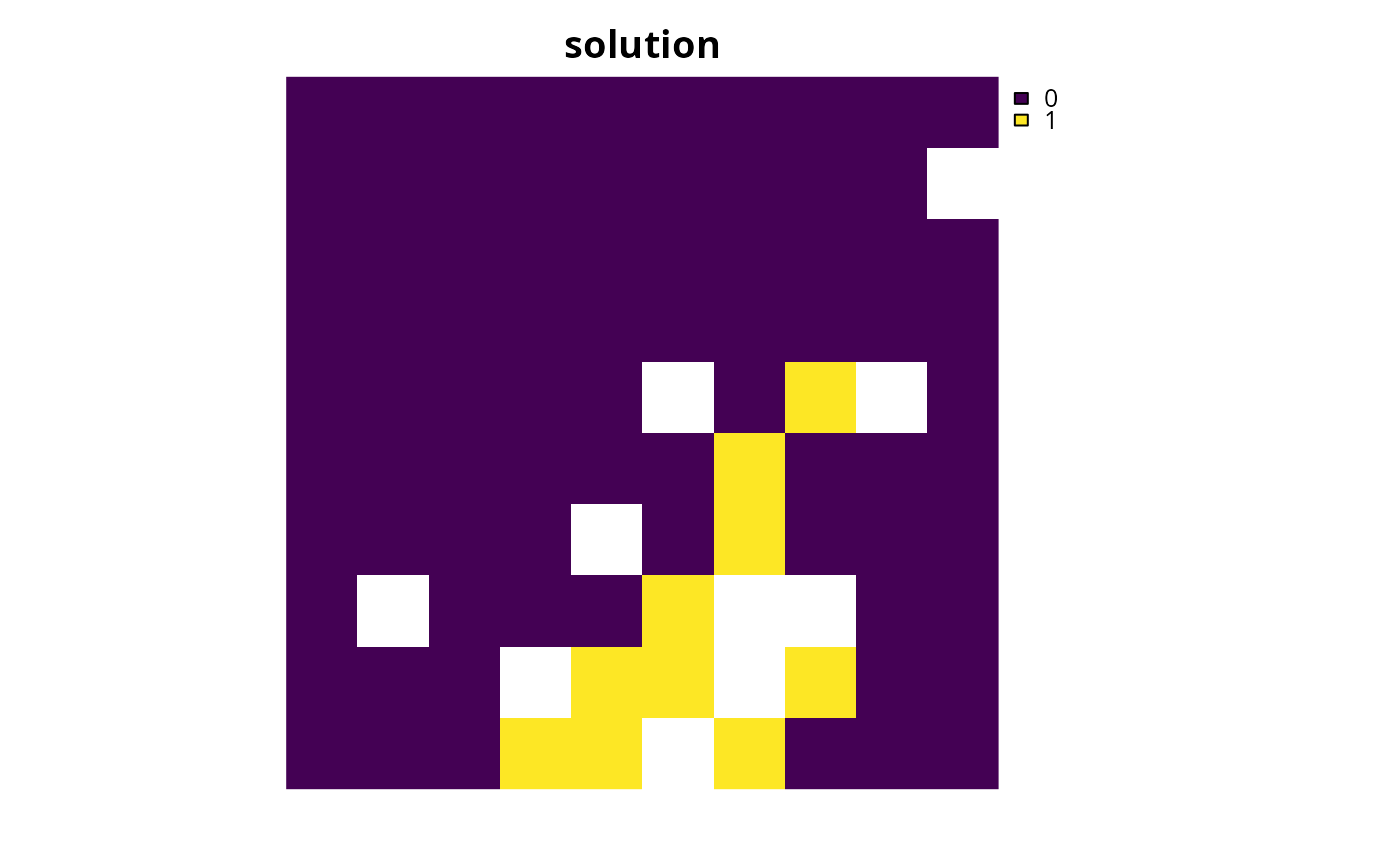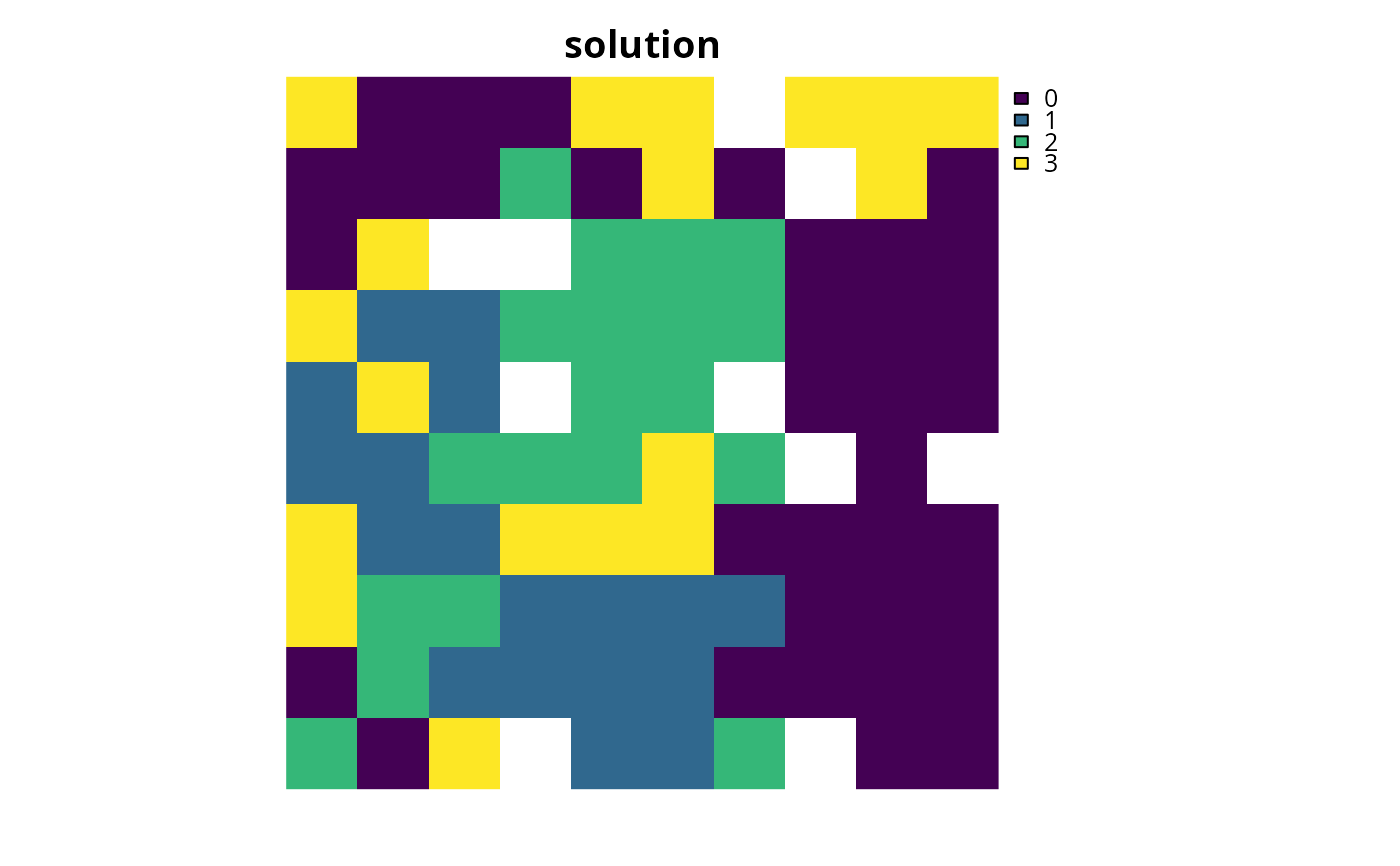Add a binary decision to a conservation planning problem. This is the classic decision of either prioritizing or not prioritizing a planning unit. Typically, this decision has the assumed action of buying the planning unit to include in a protected area network. If no decision is added to a problem then this decision class will be used by default.

## Usage

add_binary_decisions(x)

## Arguments

x

problem() object.

## Value

An updated problem() object with the decisions added to it.

## Details

Conservation planning problems involve making decisions on planning units. These decisions are then associated with actions (e.g., turning a planning unit into a protected area). Only a single decision should be added to a problem() object. Note that if multiple decisions are added to an object, then the last one to be added will be used.

See decisions for an overview of all functions for adding decisions.

Other decisions: add_proportion_decisions(), add_semicontinuous_decisions()

## Examples

# \dontrun{
# set seed for reproducibility
set.seed(500)

sim_pu_raster <- get_sim_pu_raster()
sim_features <- get_sim_features()
sim_zones_pu_raster <- get_sim_zones_pu_raster()
sim_zones_features <- get_sim_zones_features()

# create minimal problem with binary decisions
p1 <-
problem(sim_pu_raster, sim_features) %>%

# solve problem
s1 <- solve(p1)

# plot solution
plot(s1, main = "solution", axes = FALSE)# create a matrix with targets for a multi-zone conservation problem
targs <- matrix(runif(15, 0.1, 0.2), nrow = 5, ncol = 3)

# build multi-zone conservation problem with binary decisions
p2 <-
problem(sim_zones_pu_raster, sim_zones_features) %>%

# solve the problem
s2 <- solve(p2)

# print solution
print(s2)
#> class       : SpatRaster
#> dimensions  : 10, 10, 3  (nrow, ncol, nlyr)
#> resolution  : 0.1, 0.1  (x, y)
#> extent      : 0, 1, 0, 1  (xmin, xmax, ymin, ymax)
#> coord. ref. : Undefined Cartesian SRS
#> source(s)   : memory
#> names       : zone_1, zone_2, zone_3
#> min values  :      0,      0,      0
#> max values  :      1,      1,      1

# plot solution
plot(category_layer(s2), main = "solution", axes = FALSE)# }